# geometry Package¶

## geometry Package¶

This package contains definitions for the geometric primitives in use in phantomas.

## fiber Module¶

This module contains the definition of FiberSource, which is a continuous representation of a fiber. All the fibers created are supposed to connect two cortical areas. Currently, the only supported shape for the “cortical surface” is a sphere.

class phantomas.geometry.fiber.FiberSource(control_points, **kwargs)

A FiberSource is a continuous representation of a fiber trajectory,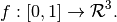The trajectory is modeled by 3 piecewise polynomials (one for each dimension). Note that the fiber is connecting two end points on the “cortical surface”. The construction makes sure that the tangents to the fiber are normal to this surface.

Parameters : control_points : array-like shape (nb_points, 3) a set of points through which the fiber will go. tangents : optional, default = ‘symmetric’ Either ‘symmetric’, ‘incoming’, ‘outgoing’. Controls the way the tangents are computed. scale : optional, default = 1.0 A multiplicative factor for the points positions. This corresponds to the sphere radius in mm.

Methods

 curvature(ts) Evaluates the curvature of the fiber at given positions. interpolate(ts) From a FiberSource, which is a continuous representation, to a tangents(ts) Get tangents (as unit vectors) at given timesteps.
curvature(ts)

Evaluates the curvature of the fiber at given positions. The curvature is computed with the formula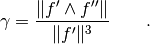Parameters : ts : array-like, shape (N, ) A list of “timesteps” between 0 and 1. curvatures : array-like, shape (N, ) The curvatures of the fiber trajectory, at selected timesteps.
interpolate(ts)

From a FiberSource, which is a continuous representation, to a Fiber, a discretization of the fiber trajectory.

Parameters : ts : array-like, shape (N, ) A list of “timesteps” between 0 and 1. trajectory : array-like, shape (N, 3) The trajectory of the fiber, discretized over the provided timesteps.
tangents(ts)

Get tangents (as unit vectors) at given timesteps.

Parameters : ts : array-like, shape (N, ) A list of “timesteps” between 0 and 1. tangents : array-like, shape (N, 3) The tangents (as unit vectors) to the fiber at selected timesteps.

## models Module¶

Model definition for the fibers geometry. A Fiber is a discrete representation of a white matter fiber bundle.

class phantomas.geometry.models.Fiber(mode, **kwargs)

A Fiber is a cylindrical shape wrapped around a discrete curve in 3D, represented by its discretization over a certain number of points.

Parameters : mode : ‘from_points’ points : array-like, shape (N, 3) A sequence of points representing the fiber. These may have been from a FiberSource. tangents : array-like, shape (N, 3) The tangents to the fiber bundle at the specified points. radius : double The radius of the fiber bundle in mm.

Methods

 get_nb_points() Gets the number of points over which the center line is defined. get_points() Gets the points of the fiber bundle centerline. get_radius() Gets the radius of the fiber bundle. intersects_bounding_box(bounding_box_extents) Computes logical intersection between current instance and given bounding box. save_to_file(index[, path]) Saves the fiber trajectory to a text file. set_radius(radius) Sets the radius of the fiber bundle.
get_nb_points()

Gets the number of points over which the center line is defined.

Returns : nb_points : int
get_points()

Gets the points of the fiber bundle centerline.

Returns : points : array-like, shape (N, 3)

Gets the radius of the fiber bundle.

intersects_bounding_box(bounding_box_extents)

Computes logical intersection between current instance and given bounding box.

Parameters : bounding_box_extents : tuple (x_min, x_max, y_min, y_max, z_min, z_max) intersects : bool
save_to_file(index, path='.')

Saves the fiber trajectory to a text file.

Parameters : index : int The identifier of the fiber bundle (will be used to format the filename). path : string The output path.

Sets the radius of the fiber bundle.

An IsotropicRegion is a spherical region defined by its radius and center. It usually defines a region of rapid diffusivity, modelling a cerebro-spinal fluid-filled region, such as ventricle.

Parameters : radius : double center : array-like, shape (3, ) The center of the spherical region.

Methods

get_center()
get_center()
Returns : center : array-like, shape (3, ) The center position of the IsotropicRegion, in real-world coordinates.
Returns : radius : double The radius of the IsotropicRegion, in mm.

## utils Module¶

This module contains utility functions for geometry.

phantomas.geometry.utils.rotation_matrix(u, v)

Given two vectors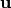and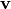, computes a rotation matrix,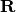, s.t.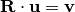.

Parameters : u : array-like, shape (3, ) v : array-like, shape (3, ) R : array-like, shape (3, 3)